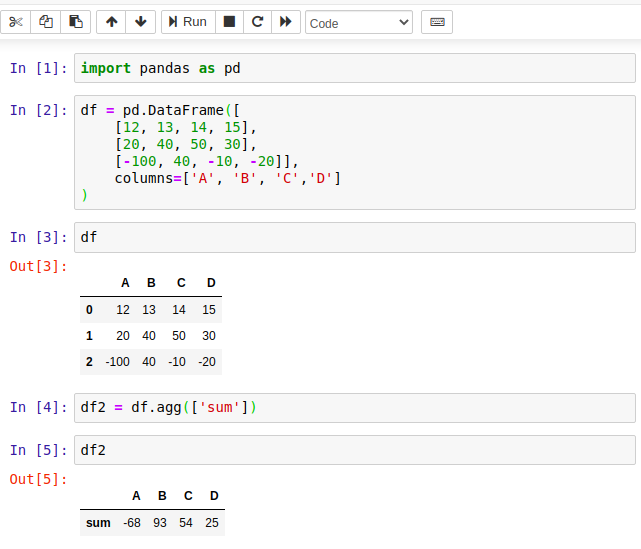# Pandas DataFrame aggregate() Method

Pandas aggregate() method is a built-in method of DataFrame that is used to perform aggregate using one or more operations over the specified axis.

We can use to apply aggregation on whole DataFrame or one or more its columns. We can pass function, string, dict, or list of functions to this method.

For example, to get the column-wise sum of all values of DataFrame we can use sum() function. Let's have some examples.

Note: agg is an alias for aggregate. So, we can use agg() in place of aggregate(), both are the same.

## Syntax

``DataFrame.aggregate(func=None, axis=0, *args, **kwargs)``

### Parameters:

func: function, str, list, or dict.

It can be a function, string function name, list of functions, or dict of functions.

axis: To specify axis, either index or columns. o represents index and 1 represents the column. The default is 0.

If 0, the function applies to each column. If 1, the function applies to each row.

*args: It represents positional arguments for func parameter.

**kwargs: Keyword arguments to pass to func parameter.

### Return Value:

It returns a scalar value, Series or DataFrame.

## Example with Jupyter Notebook

Let's take an example to understand the use of pandas.DataFrame.aggregate() method. Following is the code that is executed by using the Jupyter notebook. If you are familiar with Jupyter, See the screenshot below.## Example: How to Get the Aggregate sum of DataFrame columns in Pandas?

We can use DataFrame.aggregate() method to get sum of DataFrame columns. See the example here. This example is similar to the above code, executed by using Jupyter notebook. If you are not familiar with Jupyter then see this code to understand.

``````# import pandas library
import pandas as pd
# Create DataFrame of data
df = pd.DataFrame([
[12, 13, 14, 15],
[20, 40, 50, 30],
[-100, 40, -10, -20]],
columns=['A', 'B', 'C','D']
)
# print dataframe
print(df)
print("\nGet sum of DataFrame columns...\n")
# Sum
df2 = df.aggregate(['sum'])
print(df2)
``````

Output:

A   B   C   D
0
12  13  14  15
1   20  40  50  30
2 -100  40 -10 -20

Get sum of DataFrame columns...

A   B   C   D
sum
-68  93  54  25

## Example: Get sum and min value of DataFrame in Pandas

The DataFrame.aggregate() method can be used to get sum and min values of DataFrame columns. Here, in this example, we are using sum and min functions in the aggregate() method. It returns a DataFrame containing sum and min values. See the example here.

``````# import pandas library
import pandas as pd
# Create DataFrame of data
df = pd.DataFrame([
[12, 13, 14, 15],
[20, 40, 50, 30],
[-100, 40, -10, -20]],
columns=['A', 'B', 'C','D']
)
# print dataframe
print(df)
print("\nGet sum and min of DataFrame columns...\n")
# Sum and Min values
df2 = df.aggregate(['sum','min'])
print(df2)
``````

Output:

A   B   C   D
0   12  13  14  15
1   20  40  50  30
2 -100  40 -10 -20

Get sum and min of DataFrame columns...

A   B   C   D
sum  -68  93  54  25
min -100  13 -10 -20

## Example: Get aggreate() axis wise in Pandas

By default axis parameter is 0 for the aggregate() method and works with the DataFrame column. If we want to get a row-wise result then set axis = 1 in the DataFrame.aggregate() method. See the example here.

``````# import pandas library
import pandas as pd
# Create DataFrame of data
df = pd.DataFrame([
[12, 13, 14, 15],
[20, 40, 50, 30],
[-100, 40, -10, -20]],
columns=['A', 'B', 'C','D']
)
# print dataframe
print(df)
print("\nGet sum and min value of DataFrame rows...\n")
# Sum and Min values, row-wise
df2 = df.aggregate(['sum','min'], axis=1) # row-wise
print(df2)
``````

Output:

A   B   C   D
0
12  13  14  15
1   20  40  50  30
2 -100  40 -10 -20

Get sum and min value of DataFrame rows...

sum  min
0
54   12
1  140   20
2  -90 -100

Trending